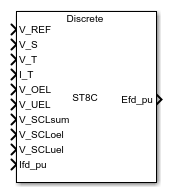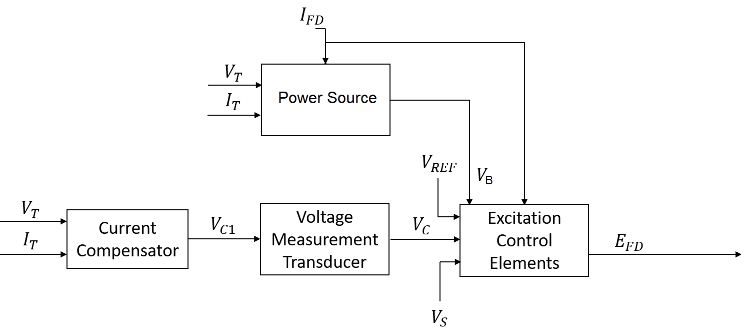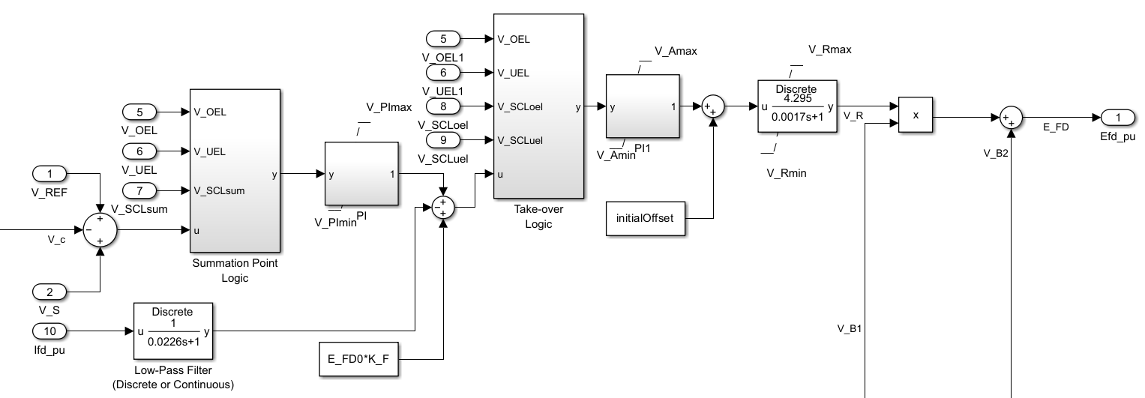# SM ST8C

Discrete-time or continuous-time synchronous machine ST8C static excitation system with automatic voltage regulator

Since R2023a

•Libraries:
Simscape / Electrical / Control / SM Control

## Description

The SM ST8C block implements a synchronous-machine-type ST8C static excitation system model in conformance with IEEE Std 421.5-2016 .

Use this block to model the control and regulation of the field voltage of a synchronous machine.

Switch between continuous and discrete implementations of the block by using the Sample time (-1 for inherited) parameter. To configure the integrator for continuous time, set the Sample time (-1 for inherited) parameter to `0`. To configure the integrator for discrete time, set the Sample time (-1 for inherited) parameter to a positive scalar. To inherit the sample time from an upstream block, set the Sample time (-1 for inherited) parameter to `-1`.

The SM ST8C block comprises four major components:

• The Current Compensator component modifies the measured terminal voltage as a function of the terminal current.

• The Voltage Measurement Transducer component simulates the dynamics of a terminal voltage transducer using a low-pass filter.

• The Excitation Control Elements component compares the voltage transducer output with a terminal voltage reference to produce a voltage error value. The component then passes this value through a voltage regulator to produce the field voltage.

• The Power Source component models the power source for the controlled rectifier when it is independent from the terminal voltage.

This diagram shows the structure of the ST8C excitation system model:In the diagram:

• VT and IT are the measured terminal voltage and current of the synchronous machine, respectively.

• VC1 is the current-compensated terminal voltage.

• VC is the filtered, current-compensated terminal voltage.

• VREF is the reference terminal voltage.

• VS is the power system stabilizer voltage.

• VB is the exciter field voltage.

• EFD and IFD are the field voltage and current, respectively.

### Current Compensator and Voltage Measurement Transducer

The block models the current compensator by using this equation:

`${V}_{C1}={V}_{T}+{I}_{T}\sqrt{{R}_{C}^{2}+{X}_{C}^{2}},$`

where:

• RC is the load compensation resistance.

• XC is the load compensation reactance.

The block implements the voltage measurement transducer as a Low-Pass Filter block with the time constant TR. Refer to the documentation for the Low-Pass Filter block for information about the exact discrete and continuous implementations.

### Excitation Control Elements

This diagram shows the structure of the excitation control elements:In the diagram:

• The Summation Point Logic subsystem models the summation point input location for the overexcitation limiter (OEL), underexcitation limiter (UEL) and stator current limiter (SCL) voltages. For more information about using limiters with this block, see Field Current Limiters.

• The Take-over Logic subsystem models the take-over point input location for the OEL, UEL, and SCL voltages. For more information about using limiters with this block, see Field Current Limiters.

• The PI subsystem models a PI controller that functions as a control structure for the automatic voltage regulator and allows the representation of an equipment retrofit with a modern digital controller. The minimum and maximum anti windup saturation limits for the block are VPImin and VPImax, respectively. A current PI regulator follows the voltage PI regulator in cascade.

• The PI1 subsystem models a PI controller that functions as a control structure for the field current regulator. The minimum and maximum anti windup saturation limits for the block are VAmin and VAmax, respectively.

• The top Low-Pass Filter block models the major dynamics of the controlled rectified bridge. KA is the controlled rectifier bridge equivalent gain and TA is the major time constant of the controlled rectifier bridge. The minimum and maximum anti windup saturation limits for the block are VRmin and VRmax, respectively.

• The Power source selector parameter controls the origin of the power source for the controlled rectifier. The contribution factor is summed and/or multiplied to the power stage output. The summation component VB2 corresponds to a compound power source derived from generator terminal current. If you set the Potential circuit (current) gain coefficient, K_I2 (pu) and Rectifier loading factor proportional to commutating reactance, K_C2 (pu) parameters to `0`, this summation component is disabled. For more information about the logical switch for the power source of the controlled rectifier, see Power Source.

### Field Current Limiters

You can use different types of field current limiter to modify the output of the voltage regulator under unsafe operating conditions:

• Use an overexcitation limiter to prevent overheating of the field winding due to excessive field current demand.

• Use an underexcitation limiter to boost field excitation when it is too low, which risks desynchronization.

• Use a stator current limiter to prevent overheating of the stator windings due to excessive current.

Attach the output of any of these limiters at one of these points:

• Summation point — Use the limiter as part of the automatic voltage regulator (AVR) feedback loop.

• Take-over point — Override the usual behavior of the AVR.

If you are using the stator current limiter at the summation point, use the input VSCLsum. If you are using the stator current limiter at the take-over point, use the overexcitation input VSCLoel, and the underexcitation input VSCLuel.

### Power Source

You can use different power source representations for the controlled rectifier by setting the Power source selector parameter value. To derive the power source for the controlled rectifier from the terminal voltage, set the Power source selector parameter to ```Position A: power source derived from generator terminal voltage```. To specify that the power source is independent of the terminal voltage, set the Power source selector parameter to ```Position B: power source independent of generator terminal conditions```.

This diagram shows a model of the exciter power source utilizing a phasor combination of the terminal voltage VT and terminal current IT:The summation component VB2 corresponds to a compound power source derived from generator terminal current. If you set the Potential circuit (current) gain coefficient, K_I2 (pu) and Rectifier loading factor proportional to commutating reactance, K_C2 (pu) parameters to `0`, this summation component is disabled.

## Ports

### Input

expand all

Voltage regulator reference set point, in per-unit representation, specified as a scalar.

Data Types: `single` | `double`

Input from the power system stabilizer, in per-unit representation, specified as a scalar.

Data Types: `single` | `double`

Terminal voltage magnitude, in per-unit representation, specified as a scalar.

Data Types: `single` | `double`

Terminal current magnitude, in per-unit representation, specified as a scalar.

Data Types: `single` | `double`

Input from the overexcitation limiter, in per-unit representation, specified as a scalar.

#### Dependencies

• To ignore the input from the overexcitation limiter, set Alternate OEL input locations (V_OEL) to `Unused`.

• To use the input from the overexcitation limiter at the summation point, set Alternate OEL input locations (V_OEL) to `Summation point`.

• To use the input from the overexcitation limiter at the take-over point, set Alternate OEL input locations (V_OEL) to `Take-over`.

Data Types: `single` | `double`

Input from the underexcitation limiter, in per-unit representation, specified as a scalar.

#### Dependencies

• To ignore the input from the underexcitation limiter, set Alternate UEL input locations (V_UEL) to `Unused`.

• To use the input from the underexcitation limiter at the summation point, set Alternate UEL input locations (V_UEL) to `Summation point`.

• To use the input from the underexcitation limiter at the take-over point, set Alternate UEL input locations (V_UEL) to `Take-over`.

Data Types: `single` | `double`

Input from the stator current limiter when using the summation point, in per-unit representation, specified as a scalar.

#### Dependencies

• To ignore the input from the stator current limiter, set Alternate SCL input locations (V_SCL) to `Unused`.

• To use the input from the stator current limiter at the summation point, set Alternate SCL input locations (V_SCL) to `Summation point`.

Data Types: `single` | `double`

Input from the stator current limiter that prevents field overexcitation when using the take-over point, in per-unit representation, specified as a scalar.

#### Dependencies

• To ignore the input from the stator current limiter, set Alternate SCL input locations (V_SCL) to `Unused`.

• To use the input from the stator current limiter at the take-over point, set Alternate SCL input locations (V_SCL) to `Take-over`.

Data Types: `single` | `double`

Input from the stator current limiter that prevents field underexcitation when using the take-over point, in per-unit representation, specified as a scalar.

#### Dependencies

• To ignore the input from the stator current limiter, set Alternate SCL input locations (V_SCL) to `Unused`.

• To use the input from the stator current limiter at the take-over point, set Alternate SCL input locations (V_SCL) to `Take-over`.

Data Types: `single` | `double`

Measured per-unit field current of the synchronous machine, specified as a scalar.

Data Types: `single` | `double`

### Output

expand all

Per-unit field voltage to apply to the field circuit of the synchronous machine, returned as a scalar.

Data Types: `single` | `double`

## Parameters

expand all

### General

Initial per-unit voltage to apply to the field circuit of the synchronous machine.

Initial per-unit voltage to apply to the terminal.

#### Dependencies

To enable this parameter, in the Exciter section, set Power source selector to ```Position A: power source derived from generator terminal voltage```.

Initial per-unit current to apply to the terminal.

#### Dependencies

To enable this parameter, in the Exciter section, set Power source selector to ```Position A: power source derived from generator terminal voltage```.

Time between consecutive block executions. During execution, the block produces outputs and, if appropriate, updates its internal state. For more information, see What Is Sample Time? and Specify Sample Time.

For inherited discrete-time operation, set this parameter to `-1`. For discrete-time operation, set this parameter to a positive integer. For continuous-time operation, set this parameter to `0`.

If this block is in a masked subsystem or a variant subsystem that supports switching between continuous operation and discrete operation, promote this parameter to ensure correct switching between the continuous and discrete implementations of the block. For more information, see Promote Block Parameters on a Mask.

### Pre-Control

Resistance used in the current compensation system. Set this parameter and Reactance component of load compensation, X_C (pu) to `0` to disable current compensation.

Reactance used in the current compensation system. Set this parameter and Resistive component of load compensation, R_C (pu) to `0` to disable current compensation.

Equivalent time constant for the voltage transducer filtering.

### Control

Per-unit proportional gain of the voltage regulator.

Per-unit integral gain of the voltage regulator.

Maximum admissible per-unit output of the PI regulator.

Minimum admissible per-unit output of the PI regulator.

Per-unit proportional gain of the field current regulator.

Per-unit integral gain of the field current regulator.

Maximum per-unit output voltage of the exciter.

Minimum per-unit output voltage of the exciter.

Gain of the controlled rectifier bridge.

Time constant of the controlled rectifier bridge.

Maximum admissible per-unit output of the field current regulator.

Minimum admissible per-unit output of the field current regulator.

Per-unit field current feedback gain of the exciter.

Feedback time constant for the stabilization of the excitation system.

Location of the overexcitation limiter input, specified as one of these options:

• `Summation point`V_OEL is an input of the Summation Point Logic subsystem.

• `Take-over`V_OEL is an input of the Take-over Logic subsystem.

Location of the underexcitation limiter input, specified as one of these options:

• `Summation point`V_UEL is an input of the Summation Point Logic subsystem.

• `Take-over`V_UEL is an input of the Take-over Logic subsystem.

Location of the stator current limiter input, specified as one of these options:

• `Summation point` — Use the V_SCLsum input port.

• `Take-over` — Use the V_SCLoel and V_SCLuel input ports.

### Exciter

Per-unit rectifier loading factor. This value is proportional to the commutating reactance.

Per-unit potential circuit gain coefficient.

Per-unit potential circuit current gain coefficient.

#### Dependencies

To enable this parameter, set Power source selector SW1 to ```Position A: power source derived from generator terminal voltage```.

Per-unit reactance associated with the potential source.

#### Dependencies

To enable this parameter, set Power source selector to ```Position A: power source derived from generator terminal voltage```.

Potential circuit phase angle, in degrees.

#### Dependencies

To enable this parameter, set Power source selector to ```Position A: power source derived from generator terminal voltage```.

Maximum per-unit available field voltage for the exciter.

Per-unit loading factor of the rectifier. This value is proportional to the commutating reactance, derived from the generator terminal current through a separate series diode bridge.

Per-unit compound circuit current gain coefficient derived from the generator terminal current through a separate series diode bridge. Set this parameter to `0` to disable the separate compound power source completely.

Maximum per-unit available field voltage for the exciter, derived from the generator terminal current through a separate series diode bridge.

Position of the power source selector.

 IEEE Std 421.5-2016 (Revision of IEEE Std 421.5-2005). "IEEE Recommended Practice for Excitation System Models for Power System Stability Studies." Piscataway, NJ: IEEE, 2016.